Full Text:<4748>

CLC number: TU45

On-line Access: 2013-01-02

Revision Accepted: 2012-11-15

Crosschecked: 2012-12-10

Cited: 7

Clicked: 6575

Citations:  Bibtex RefMan EndNote GB/T7714

 Journal of Zhejiang University SCIENCE A 2013 Vol.14 No.1 P.61-70 http://doi.org/10.1631/jzus.A1200167

Analytical solution for 1D consolidation of unsaturated soil with mixed boundary condition

 Author(s):  Zhen-dong Shan1,2, Dao-sheng Ling1, Hao-jiang Ding1 Affiliation(s):  1. MOE Key Lab of Soft Soils and Geo-environmental Engineering, Zhejiang University, Hangzhou 310058, China; more Corresponding email(s):   dsling@zju.edu.cn Key Words:  Unsaturated soil, Consolidation, Mixed boundary condition, Analytical solution Share this article to： More <<< Previous Article|Next Article >>>

Zhen-dong Shan, Dao-sheng Ling, Hao-jiang Ding. Analytical solution for 1D consolidation of unsaturated soil with mixed boundary condition[J]. Journal of Zhejiang University Science A, 2013, 14(1): 61-70.

@article{title="Analytical solution for 1D consolidation of unsaturated soil with mixed boundary condition",
author="Zhen-dong Shan, Dao-sheng Ling, Hao-jiang Ding",
journal="Journal of Zhejiang University Science A",
volume="14",
number="1",
pages="61-70",
year="2013",
publisher="Zhejiang University Press & Springer",
doi="10.1631/jzus.A1200167"
}

%0 Journal Article
%T Analytical solution for 1D consolidation of unsaturated soil with mixed boundary condition
%A Zhen-dong Shan
%A Dao-sheng Ling
%A Hao-jiang Ding
%J Journal of Zhejiang University SCIENCE A
%V 14
%N 1
%P 61-70
%@ 1673-565X
%D 2013
%I Zhejiang University Press & Springer
%DOI 10.1631/jzus.A1200167

TY - JOUR
T1 - Analytical solution for 1D consolidation of unsaturated soil with mixed boundary condition
A1 - Zhen-dong Shan
A1 - Dao-sheng Ling
A1 - Hao-jiang Ding
J0 - Journal of Zhejiang University Science A
VL - 14
IS - 1
SP - 61
EP - 70
%@ 1673-565X
Y1 - 2013
PB - Zhejiang University Press & Springer
ER -
DOI - 10.1631/jzus.A1200167

Abstract:
Based on consolidation equations proposed for unsaturated soil, an analytical solution for 1D consolidation of an unsaturated single-layer soil with nonhomogeneous mixed boundary condition is developed. The mixed boundary condition can be used for special applications, such as tests occur in laboratory. The analytical solution is obtained by assuming all material parameters remain constant during consolidation. In the derivation of the analytical solution, the nonhomogeneous boundary condition is first transformed into a homogeneous boundary condition. Then, the eigenfunction and eigenvalue are derived according to the consolidation equations and the new boundary condition. Finally, using the method of undetermined coefficients and the orthogonal relation of the eigenfunction, the analytical solution for the new boundary condition is obtained. The present method is applicable to various types of boundary conditions. Several numerical examples are provided to investigate the consolidation behavior of an unsaturated single-layer soil with mixed boundary condition.

## 1.  Introduction

The application of a load to an unsaturated soil specimen will result in the generation of excess pore-air and pore-water pressures. The excess pore pressures will dissipate with time and eventually return to their initial values. The dissipation processes of excess pore pressures are called consolidation and result in a volume decrease (Fredlund and Rahardjo, ). Many different consolidation equations have been proposed to describe the consolidation behavior of unsaturated soil. Biot () and Scott () proposed consolidation equations for unsaturated soil with occluded air bubbles. Blight () derived a consolidation equation for the air phase of a dry, rigid, and unsaturated soil. Barden (; ) presented an analysis of the consolidation of compacted and unsaturated clay. Assuming that the air and water phases are continuous, Fredlund and Hasan () proposed a 1D consolidation theory, now widely accepted, in which two partial differential equations are employed to describe the dissipation processes of excess pore pressures in unsaturated soil. This theory was later extended to the 3D case by Dakshanamurthy et al. ().

The consolidation equation for saturated soil proposed by Terzaghi () is a linear equation, while the consolidation equations given by Fredlund and Hasan () and Dakshanamurthy et al. () are nonlinear. Therefore, the consolidation problems for unsaturated soil are solved via integral transform method or numerical methods involving the discretisation of both spatial and temporal domains (Wong et al., ; Conte, ; ; Geng et al., ). For simplicity, assuming all the soil parameters remain constant during consolidation, Fredlund and Rahardjo () presented 1D consolidation equations in the form of linear equations.

Using the simplified consolidation equations, several analytical solutions for the 1D consolidation of unsaturated soil have been published. Qin et al. () adopted the Laplace transform method and gave an analytical solution for an unsaturated single-layer soil subjected to a large-area uniform load, with the top surface being permeable and the bottom surface being impermeable to air and water. Qin et al. () obtained an analytical solution for unsaturated single-layer soil subjected to a gradually-increasing load using the same method and employing the same boundary condition as Qin et al. (). Shan et al. () used the separation of variables method to give exact solutions for unsaturated single-layer soil subjected to an arbitrary load and with three types of boundary conditions.

These analytical solutions are for unsaturated single-layer soil with boundaries that are permeable or impermeable to both air and water phases. However, lab-testing equipment is commonly designed such that air flows upwards and water flows downwards during a test, i.e., the top boundary is permeable to air and impermeable to water, while the bottom boundary has the reverse condition. Fredlund and Rahardjo () named this type of boundary condition a mixed boundary condition, the analytical solution for which has not been published.

As an extension of Shan et al. (), this paper presents an analytical method which can be used to solve various kinds of boundary value problems. As an example, an analytical solution for an unsaturated single-layer soil with mixed boundary condition is presented. Note that the material parameters in the consolidation equations are assumed to be constant during consolidation. This state may not be practical. However, the analytical solution of the simplified consolidation equation gives a preliminary description of the dissipation laws of the air and water pressures, and enables the study of the evolution law of the volume change of an unsaturated single-layer soil. Moreover, the analytical solution can be used to validate the accuracy of various numerical results. Section 2 outlines the consolidation equations for unsaturated soil, as proposed by Fredlund and Hasan (). The associated initial condition and mixed boundary condition are imposed in section 3. In section 4, the derivation of the analytical solution is presented in detail. In section 5, several examples are given to illustrate some interesting features of unsaturated single-layer soil with mixed boundary condition.

## 2.  Consolidation equations for unsaturated soil

Fredlund and Hasan () developed the basic equations for the 1D consolidation of unsaturated soil in the Cartesian coordinate system, based on the assumption that the air and water flows are continuous and interdependent. These equations are employed here to study the consolidation behavior of unsaturated soil. Assuming all variables depend on the z direction only, the consolidation equations are: , where where u w and u a represent the excess pore-water and pore-air pressures, respectively, and q(t) is the external load. In addition where k w is the water permeability coefficient, k a is the air permeability coefficient and g is the acceleration due to gravity. and are the coefficients of the water and air volume changes, respectively, due to the net normal stress σu a for a K 0-load condition; and are the coefficients of the water and air volume changes, respectively, due to the matrix suction u au w. σ is the total stress, S is the saturation of water, n is the porosity, γ w is the unit weight of water, and ω a is the molecular mass of air. is the absolute pore-air pressure which can be expressed as , where is the atmospheric pressure. When u a is small or dissipates rapidly in the process of consolidation, can be considered to be constant (Conte, ), and let in this study. R is the universal gas constant, and T is the absolute temperature which can be expressed as T=(t+273.16) K, where t is the temperature, °C.

The compression of unsaturated single-layer soil (Shan et al., ) can be obtained by , where H is the thickness of the layer, and . If the external load is constant, Eq. (4) can be simplified as the expression for compression given by Ausilio and Conte ().

## 3.  Initial and boundary conditions

To address the consolidation of an unsaturated single-layer soil (Fig. 1), associated initial and boundary conditions must be imposed. The following initial condition is employed: , where g (z)={g 1(z), g 2(z)}T, with g 1(z) and g 2(z) being arbitrarily specified functions.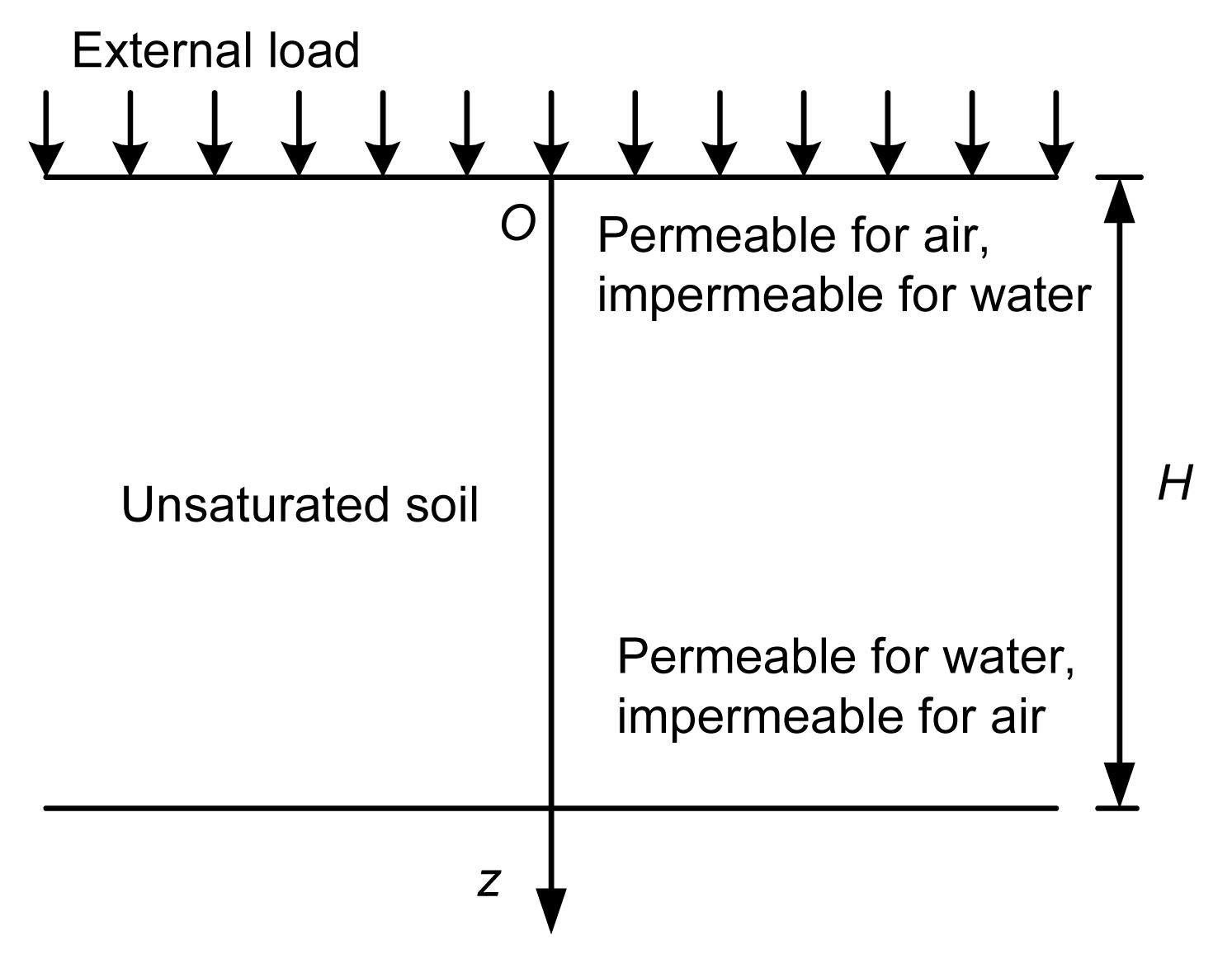Fig.1
An unsaturated single-layer soil with mixed boundary condition

The following boundary condition is considered: where fj (t) (j=1, 2, 3, 4) are arbitrarily specified functions. When fj (t) (j=1, 2, 3, 4) is equal to zero, boundary condition Eq. (6) becomes the mixed boundary condition as proposed by Fredlund and Rahardjo ().

## 4.  Derivation of the analytical solution

The method of Shan et al. () can address problems with three types of boundary conditions, but cannot solve problems with mixed boundary conditions. By incorporating some improvements to the method of Shan et al. (), the present method can be used to solve various kinds of boundary value problems. The solution procedure is illustrated below.

### 4.1.  Transformation of nonhomogeneous boundary conditions

Boundary condition Eq. (6) is nonhomogeneous, while the traditional eigenfunction expansion method can deal only with problems with homogeneous boundary conditions. To solve Eq. (1) subject to boundary condition Eq. (6) and initial condition Eq. (5), the vector u is divided into , where u s is a function which is specified to satisfy the nonhomogeneous boundary condition Eq. (6), and can be chosen simply as .

By substituting Eq. (7) into Eq. (1), initial condition Eq. (5), and boundary condition Eq. (6), the governing equation in terms of u d is obtained as follows: , which is subject to the following initial condition and homogeneous boundary condition: ,

Thus, the nonhomogeneous boundary value problem (i.e., Eq. (1) subject to initial condition Eq. (5) and boundary condition Eq. (6)) has been successfully transformed into a homogeneous boundary value problem (i.e., Eq. (9) subject to initial condition Eq. (10) and boundary condition Eq. (11)), which can be solved by the eigenfunction expansion method.

### 4.2.  Eigenfunction and eigenvalue

To solve the nonhomogeneous partial differential Eq. (9), the following characteristic equation is first considered to derive the corresponding eigenfunction and eigenvalue: .

The solution of Eq. (12) has the following form: , where , and ω is the eigenvalue which is a non-negative real number. By substituting Eq. (13) into Eq. (12) and boundary condition Eq. (11), we obtain ,

The solution of Eq. (14) under Eq. (15) will be described when ω=0 and ω>0.

When ω=0, Eq. (14) can be simplified as . As the determinant of matrix K is not equal to zero, the general solution of Eq. (16) is as follows: , where c 01, c 02, d 01, and d 02 are constants. By substituting Eq. (17) into boundary condition Eq. (15), these constants in Eq. (17) can be determined, and thus we can obtain: , which can be eliminated.

When ω>0, the general solution of Eq. (14) can be chosen simply as , where β d is an unknown constant, and F is an undetermined second-order vector. Substituting Eq. (19) into Eq. (14), the following equation in terms of F is obtained: . If, and only if, the determinant of the above coefficient matrix is equal to zero, Eq. (20) has a nonzero solution, and thus we obtain the following equation in terms of β d: , To solve Eq. (21), we first obtain the two expressions of y 2, labeled A and B, respectively, i.e., . According to the signs (positive or negative) and the size relation of the constants Δ, b, and c, the solution y of Eq. (21) has different expressions, which should be discussed in detail from a mathematical point of view. The case Δ>0, c>0, and b>0 is studied in this paper because most unsaturated soils satisfy this situation, including the five groups of material parameters of Kaolin soil described by Fredlund and Rahardjo (). For other cases, the solution y can be obtained using the same method.

When Δ>0, c>0, and b<0, we know that A<0 and B<0; thus, the four roots of Eq. (21) can be written as . Substituting yi (j=1, 2, 3, 4) into the first equation of Eq. (22), β dj is first obtained. Then, by substituting β dj into Eq. (20), the corresponding F j can be obtained as follows: , where Aj (j=1, 2, 3, 4) are constants, and . Therefore, the solution of Eq. (14) can be written as If, and only if, Aj are conjugate complex numbers, X (z) is a real function. We thus set A 1 =(b 1−ib 2)/2, A 2 =(b 3−ib 4)/2, and . Eq. (27) can be transformed into: , where ω>0 and bj (j=1, 2, ,3, 4) are real numbers.

At this point, the eigenfunction of Eq. (9) under boundary condition Eq. (11) has been obtained as shown in Eqs. (18) and (28). As the next step, we start to determine the corresponding eigenvalue through boundary condition Eq. (15). By substituting Eq. (28) into Eq. (15), we obtain the following homogeneous system of linear equations: , where , , , , , , , , , , , . If, and only if, the determinant of the coefficient matrix is equal to zero, Eq. (29) has a nonzero solution, and thus we obtain the characteristic equation of &ohgr; as follows: . This is a transcendental equation which has infinite positive roots labeled ωk (k=1, 2, …) from the smallest to the largest, respectively.

Using Eq. (29), a set of b 1, b 2, b 3, and b 4 can be obtained as follows: where the subscript k indicates eigenvalue ωk . Considering Eqs. (18) and Eq. (28), the eigenfunction of Eq. (9) under boundary condition Eq. (11) can be written as , where the eignvalue ωk is determined by Eq. (30).

### 4.3.  Orthogonality of the eigenfunction

In this section, we demonstrate the orthogonality of the eigenfunction, which will be used in the next section. Let &ohgr;p and &ohgr;q be eigenvalues and and the corresponding eigenfunctions. Using Eq. (12), the following two equations are obtained: , . By subtracting Eq. (33b) from Eq. (33a), integrating Eq. (33a with respect to z from 0 to H, then using the symmetry of matrix C , we obtain . By using the method of integration by parts and the symmetry of matrix K , the first integration in Eq. (34) can be rewritten as Substituting boundary conditions Eq. (15) into Eq. (35), we know Eq. (35) equals zero. Eq. (34) can thus be simplified as follows: which indicates that is orthogonal to with respect to matrix C , where Gp is a constant which can be expressed as , where .

### 4.4.  Analytical solution of the nonhomogeneous partial differential equation

As the last step, we solve Eq. (9). By using the method of undetermined coefficients and the principle of linear superposition, the solution of Eq. (9) subject to initial condition Eq. (10) and boundary condition Eq. (11) can be written as , where are shown as Eq. (32). Tk (t) are undetermined scalar functions. Substituting Eq. (38) into Eqs. (9) and (10), we have , . Eqs. (39) and (40) can be simplified through the orthogonality of as shown in Eq. (36). Pre-multiplying Eqs. (39) and (40) by , and then integrating Eqs. (39) and (40) with respect to z from 0 to H, we obtain , , where . The solution of Eq. (41) under initial condition Eq. (42) is given as follows: . By substituting Eq. (44) into Eq. (38), u d(z, t) can be obtained. Substituting u d(z, t) and u s(z, t) into Eq. (7), the expression of u (z, t) is finally obtained.

## 5.  Numerical examples

All examples in this section adopt the following parameters (Qin et al., ): and the following necessary parameters: where q 0 is the amplitude of the external load.

It is assumed that the total stress σ(z, t) in unsaturated soil is zero before external excitation is applied to the layer, and the initial conditions are as follows: . Note that both u w and u a are excess pore pressures. Assume the external excitation is a step load or an exponential load, as shown in Fig. 2, and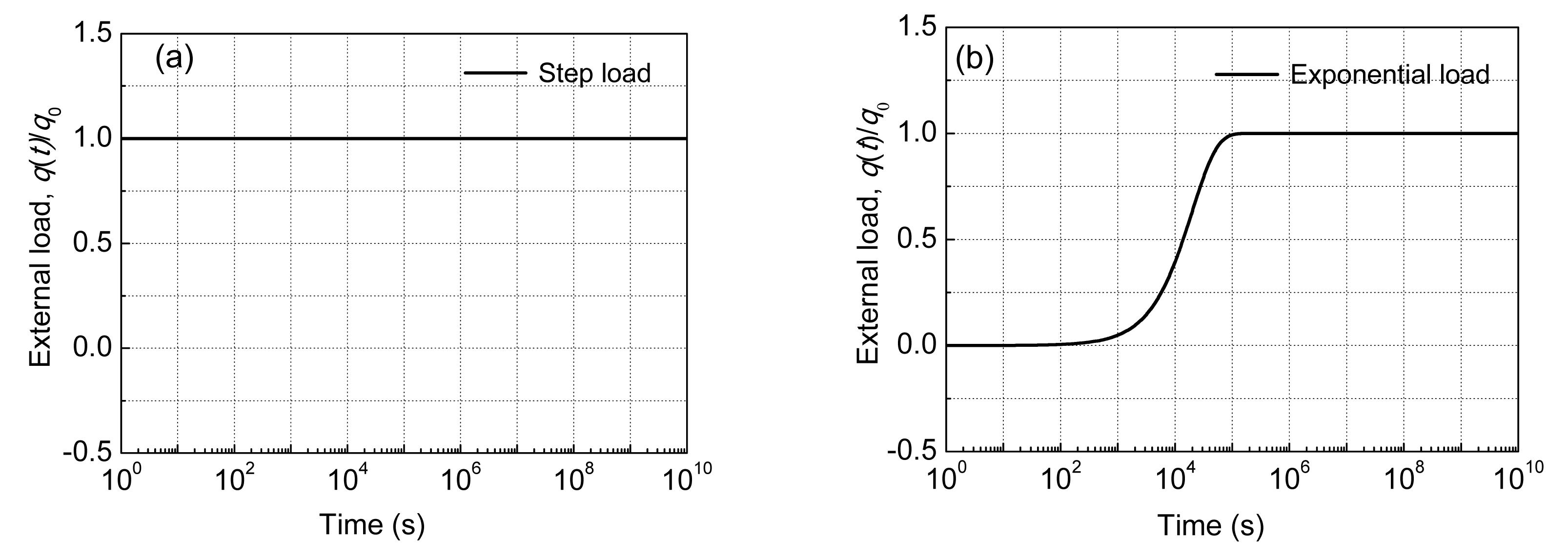Fig.2

Assume an unsaturated single-layer soil is subjected to a step load and has the following boundary condition: Boundary condition Eq. (50) indicates that the top boundary is permeable to air and impermeable to water, while the bottom boundary has the reverse condition.

Fig. 3 shows the variation in the excess pore-air and pore-water pressures over time in z=5 m due to a step load. The application of the step load to the unsaturated single-layer soil results in the generation of excess pore-air and pore-water pressures. The excess pore pressures dissipate with time and eventually return to zero, and the dissipation of u a is much faster than that of u w. Also, the u w dissipation curves show major differences for different k a, which means that variation in u a has a significant influence on that of u w. This is mainly because the dissipation of u a is much faster than that of u w.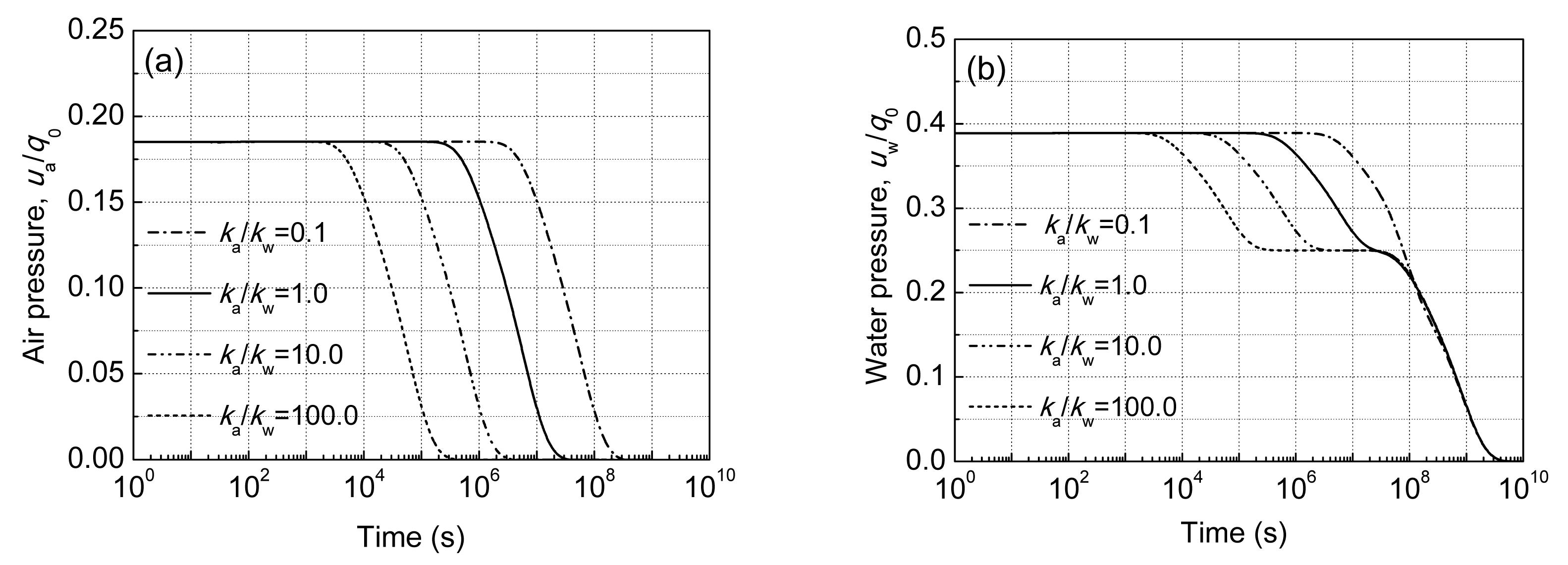Fig.3
Excess pore-air pressure (a) and pore-water pressure (b) over time in z=5 m

Fig. 4 illustrates how the excess pore-air and pore-water pressures vary with depth when k a/k w=1. The dissipation of u a starts from the top part of the layer and gradually extends to the bottom part, and u a eventually returns to zero. However, the dissipation of u w can be divided into two phases (Fig. 4). Due to the influence of u a, the decrease in u w in the top part is faster than that in the bottom part. But, after u a is diminished, the dissipation of u w in the bottom part is faster than that in the top part.Fig.4
Excess pore-air pressure (a) and pore-water pressure (b) over depth at different times

Fig. 5 shows the variation in the excess pore-air and pore-water pressures over time in the middle of the layer for different water saturations, when k a/k w=1. Instantaneous excess pore-air and pore-water pressure increases occur at the time when the step load is applied. Moreover, these instantaneous increases are larger at higher water saturation levels.Fig.5
Excess pore-air pressure (a) and pore-water pressure (b) over time for different water saturation levels

Assume an unsaturated single-layer soil is restricted by boundary condition Eq. (50) and subjected to an exponential load as shown in Eq. (49). Fig. 6 shows the variation in the excess pore-air and pore-water pressures over time in z=5 m. The application of the exponential load results in gradual increases in u w and u a. When the external load tends to be constant, the excess pore pressures no longer increase but gradually decrease with time, eventually returning to zero.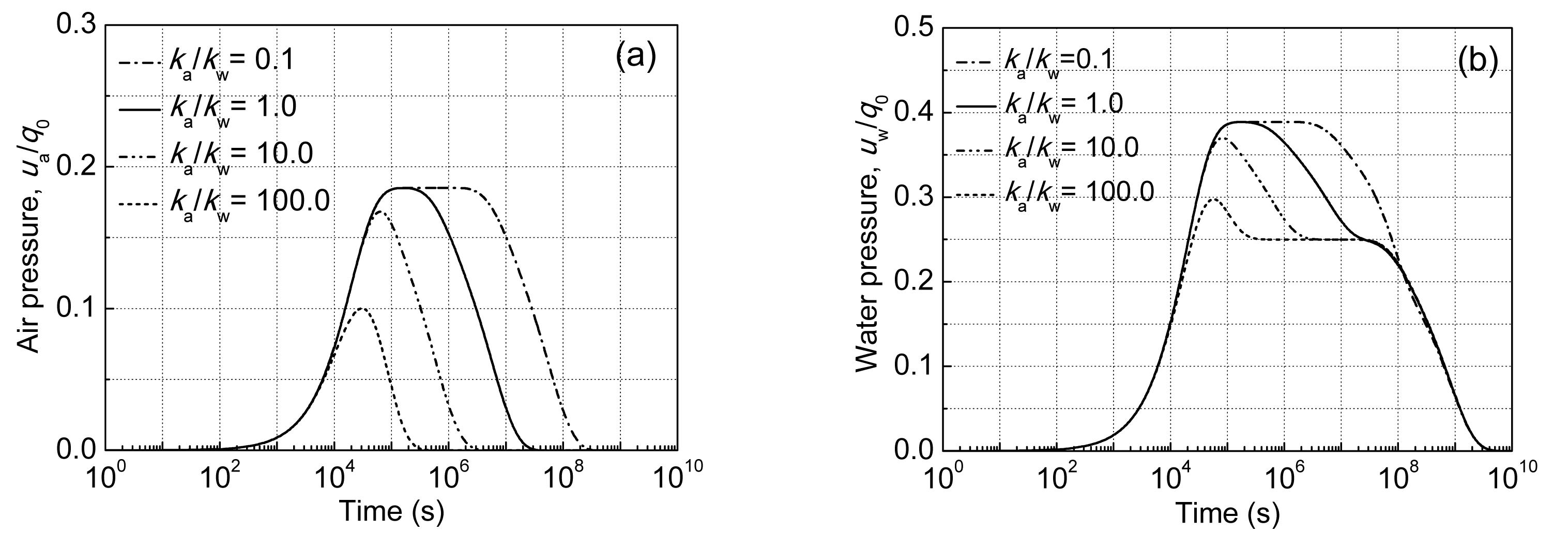Fig.6
Excess pore-air and pore-water pressures over time in z=5 m

Fig. 7 shows typical plots of compressions of an unsaturated single-layer soil due to two different loads, where . The application of a step load (Fig. 7a) results in a “significant immediate compression”. The compression of the layer then gradually increases and eventually becomes constant. If an unsaturated single-layer soil is subjected to an exponential load (Fig. 7b), the compression of the layer gradually goes up with the rise in the external load. When the external load becomes constant and the excess pore pressures are diminished, the compression becomes constant.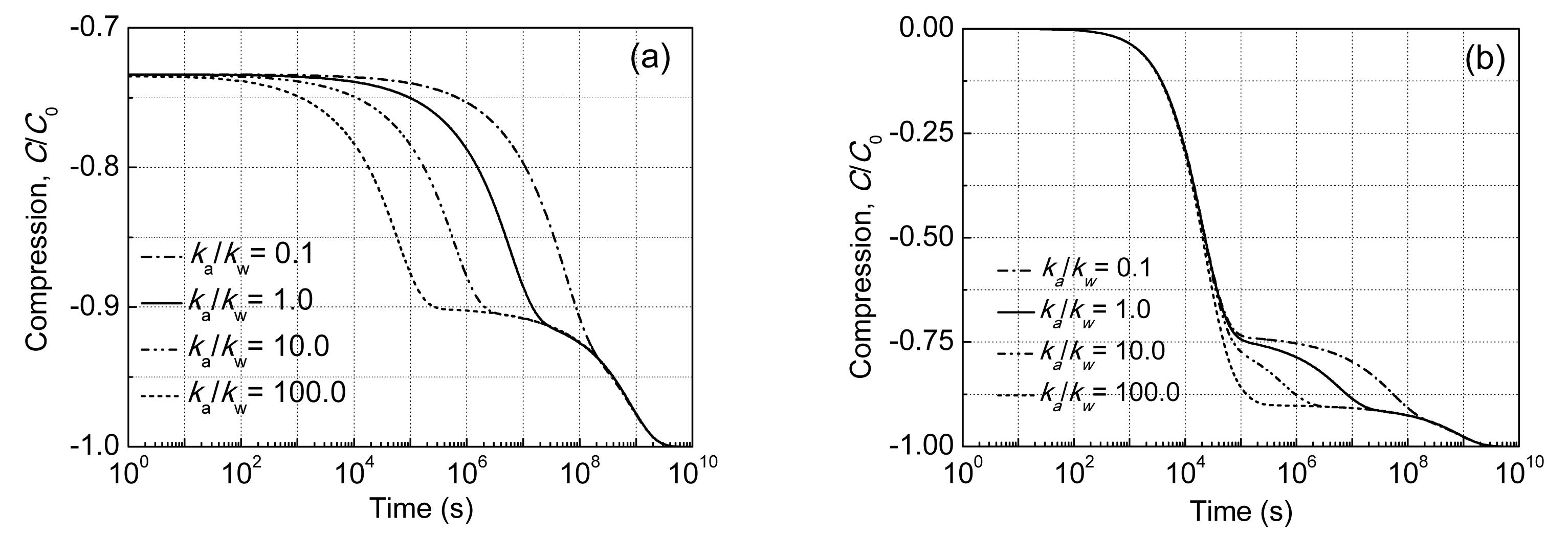Fig.7
Compressions of the unsaturated single-layer soil under step load (a) and exponential load (b)

### 5.3.  External air pressure

Assume an unsaturated single-layer soil is subjected to a step load and has the following boundary condition:

Boundary condition Eq. (51) means that the air pressure on the top boundary changes as a sine wave with time, and Ω denotes the frequency.

Fig. 8 shows how the excess pore-air and pore-water pressures vary with time in z=5 m due to a step load. Comparison of Fig. 8 and Fig. 3 shows the influence of the excess pore-air pressure on the boundary on the excess pore-air and pore-water pressures in unsaturated soil. The variation in the excess pore-air pressure on the boundary has a great influence on the excess pore-air and pore-water pressures in unsaturated soil. This is due mainly to the fast spread of air in unsaturated soil.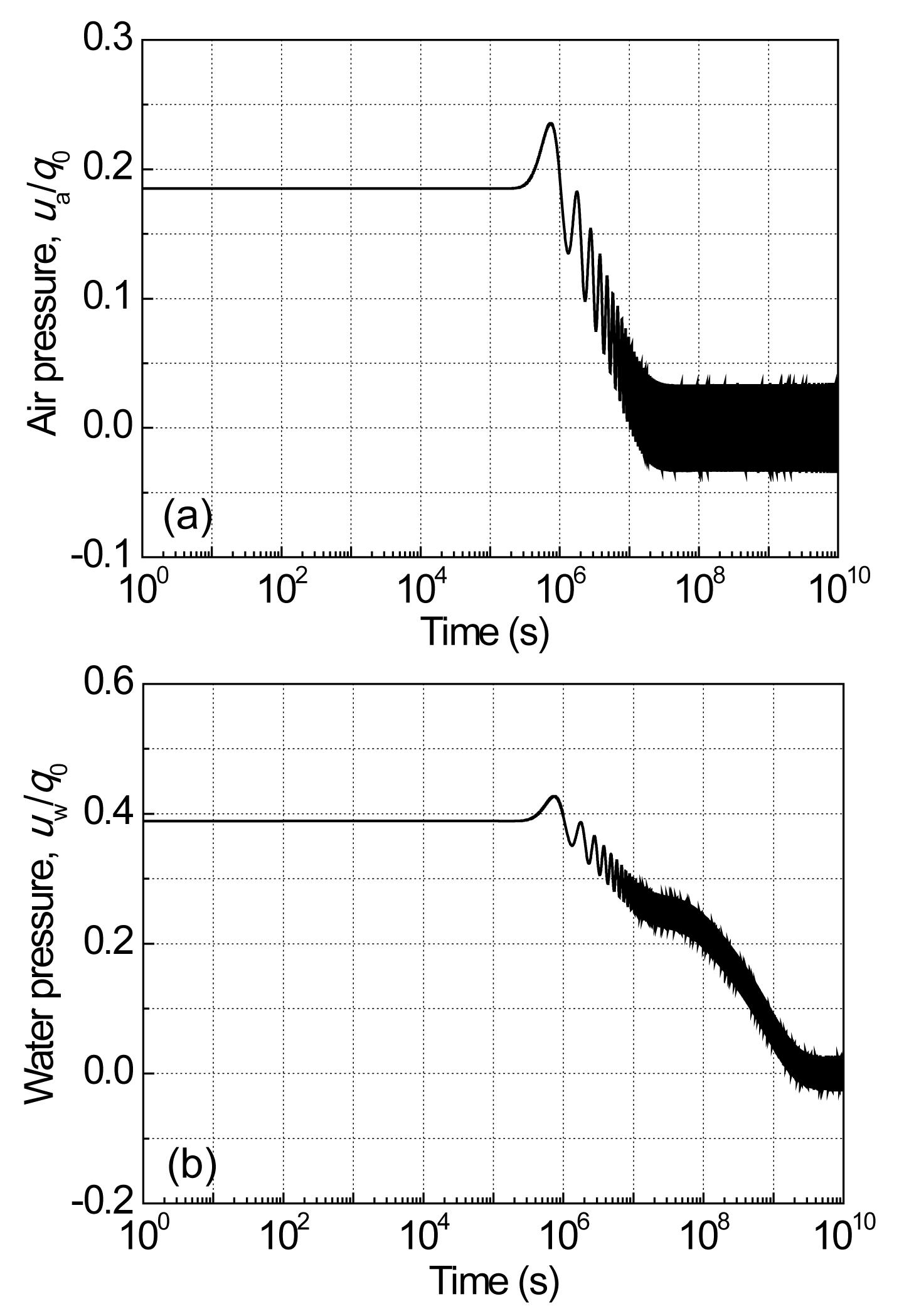Fig.8
Excess pore-air pressure (a) and pore-water pressure (b) over time in z=5 m with Ω=2×10−6

## 6.  Conclusions

The 1D consolidation of unsaturated single-layer soil with mixed boundary condition was studied based on the consolidation equations proposed by Fredlund and Hasan (), in which the material parameters are assumed to be constants during consolidation. The method described represents an extension of the method of Shan et al. () and can be used to solve various kinds of boundary conditions. An analytical solution for an unsaturated single-layer soil subjected to an arbitrary load was also obtained. The analytical solution reveals the dissipation rules of the excess pore-air and pore-water pressures, and can be used to validate the accuracy of various numerical results. The consolidation behavior of unsaturated soil was investigated through several numerical examples. We conclude that the dissipation of excess pore-air pressure is much faster than that of excess pore-water pressure, and that variation in the excess pore-air pressure has a great influence on that of excess pore-water pressure.

## ReferencesAusilio, E., Conte, E., 1999. Settlement rate of foundations on unsaturated soils. Canadian Geotechnical Journal, 36(5):940-946.Barden, L., 1965. Consolidation of compacted and unsaturated clays. Gotechnique, 15(3):267-286.Barden, L., 1974. Consolidation of clays compacted dry and wet of optimum water content. Gotechnique, 24(4):605-625.Biot, M.A., 1941. General theory of three-dimensional consolidation. Journal of Applied Physics, 12(2):155-164.Blight, G.E., 1961.  Strength and Consolidation Characteristics of Compacted Soils. PhD Thesis, University of London,England :Conte, E., 2004. Consolidation analysis for unsaturated soils. Canadian Geotechnical Journal, 41(4):599-612.Conte, E., 2006. Plane strain and axially symmetric consolidation in unsaturated soils. International Journal of Geomechanics, 6(2):131-135.Dakshanamurthy, V.V., Fredlund, D.G., Rahardjo, H., 1984. Coupled Three-Dimensional Consolidation Theory of Unsaturated Porous Media. , Fifth International Conference on Expansive Soils, Adelaide, South Australia, 99-103. :99-103.Fredlund, D.G., Hasan, J.U., 1979. One-dimensional consolidation theory: unsaturated soils. Canadian Geotechnical Journal, 16(3):521-531.Fredlund, D.G., Rahardjo, H., 1993.  Soil Mechanics for Unsaturated Soil. John Wiley and Sons,New York :Geng, X.Y., Xu, C.J., Cai, Y.Q., 2006. Non-linear consolidation analysis of soil with variable compressibility and permeability under cyclic loads. International Journal for Numerical and Analytical Methods in Geomechanics, 30(8):803-821.Qin, A.F., Chen, G.J., Tan, Y.W., Sun, D.A., 2008. Analytical solution to one-dimensional consolidation in unsaturated soils. Applied Mathematics and Mechanics, 29(10):1329-1340.Qin, A.F., Sun, D.A., Tan, Y.W., 2010. Analytical solution to one-dimensional consolidation in unsaturated soils under load varying exponentially with time. Computers and Geotechnics, 37(1-2):233-238.Scott, R.F., 1963.  Principles of Soil Mechanics. Addison-Wesley Publishing Company, Inc,Reading, MA :Shan, Z.D., Ling, D.S., Ding, H.J., 2012. Exact solutions for one dimensional consolidation of single layer unsaturated soil. International Journal for Numerical and Analytical Methods in Geomechanics, 36(6):708-722.Terzaghi, K., 1943.  Theoretical Soil Mechanics. Wiley,New York :Wong, T.T., Fredlund, D.G., Krahn, J., 1998. A numerical study of coupled consolidation in unsaturated soils. Canadian Geotechnical Journal, 35(6):926-937.